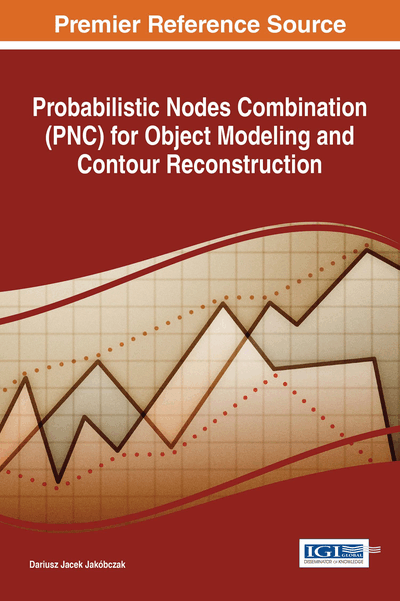# Contour Reconstruction: 2D Object Modeling

DOI: 10.4018/978-1-5225-2531-8.ch004
Available
\$37.50
No Current Special Offers

## Abstract

The method of Probabilistic Features Combination (PFC) enables interpolation and modeling of high-dimensional N data using features' combinations and different coefficients ?: polynomial, sinusoidal, cosinusoidal, tangent, cotangent, logarithmic, exponential, arc sin, arc cos, arc tan, arc cot or power function. Functions for ? calculations are chosen individually at each data modeling and it is treated as N-dimensional probability distribution function: ? depends on initial requirements and features' specifications. PFC method leads to data interpolation as handwriting or signature identification and image retrieval via discrete set of feature vectors in N-dimensional feature space. So PFC method makes possible the combination of two important problems: interpolation and modeling in a matter of image retrieval or writer identification. Main features of PFC method are: PFC interpolation develops a linear interpolation in multidimensional feature spaces into other functions as N-dimensional probability distribution functions.
Chapter Preview
Top

## Introduction

Probabilistic modeling is still developing branch of the computer science: operational research (for example probabilistic model-based prognosis) (Lorton, Fouladirad & Grall, 2013), decision making techniques and probabilistic modeling (Pergler & Freeman, 2008), artificial intelligence and machine learning. There are used different aspects of probabilistic methods: stochastic processes and stochastic model-based techniques, Markov processes (Cocozza-Thivent, Eymard, Mercier & Roussignol, 2006), Poisson processes, Gamma processes, a Monte Carlo method, Bayes rule, conditional probability and many probability distributions. In this chapter the goal of probability distribution function is to describe the position of unknown points between given interpolation nodes. Two-dimensional curve (opened or closed) is used to represent the data points.

So problem statement of this chapter is: how to reconstruct (interpolate) missing points of 2D curve having the set of interpolation nodes (key points) and using the information about probabilistic distribution of unknown points. For example the simplest basic distribution leads to the easiest interpolation – linear interpolation. Apart from probability distribution, additionally there is the second factor of proposed interpolation method: nodes combination. The simplest nodes combination is zero. Thus proposed curve modeling is based on two agents: probability distribution and nodes combination.

## Complete Chapter List

Search this Book:
Reset